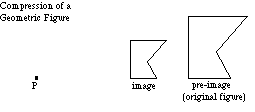index: click on a letter A B C D E F G H I J K L M N O P Q R S T U V W X Y Z A to Z index index: subject areas numbers & symbols sets, logic, proofs geometry algebra trigonometry advanced algebra & pre-calculus calculus advanced topics probability & statistics real world applications multimedia entrieswww.mathwords.com about mathwords website feedback

 Compression of a Geometric Figure Contraction of a Geometric Figure A transformation in which all distances are shortened by a common factor. This is done by contracting all points toward some fixed point P. Note: The common factor is less than 1 for a contraction. When the common factor is greater than 1 the transformation is called a dilation.See also